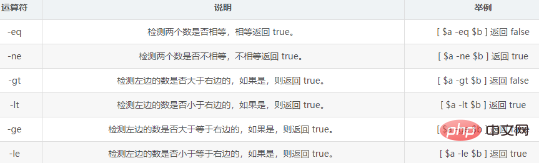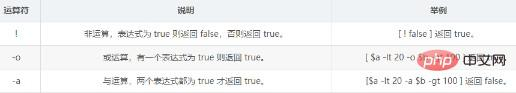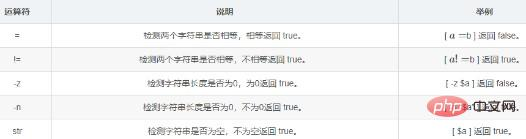﻿ shell关系运算符介绍 - 自由资讯

# shell关系运算符介绍```#!/bin/bash

a=10
b=20

if [ \$a -eq \$b ]
then
echo "\$a -eq \$b : a 等于 b"
else
echo "\$a -eq \$b: a 不等于 b"
fi
if [ \$a -ne \$b ]
then
echo "\$a -ne \$b: a 不等于 b"
else
echo "\$a -ne \$b : a 等于 b"
fi
if [ \$a -gt \$b ]
then
echo "\$a -gt \$b: a 大于 b"
else
echo "\$a -gt \$b: a 不大于 b"
fi
if [ \$a -lt \$b ]
then
echo "\$a -lt \$b: a 小于 b"
else
echo "\$a -lt \$b: a 不小于 b"
fi
if [ \$a -ge \$b ]
then
echo "\$a -ge \$b: a 大于或等于 b"
else
echo "\$a -ge \$b: a 小于 b"
fi
if [ \$a -le \$b ]
then
echo "\$a -le \$b: a 小于或等于 b"
else
echo "\$a -le \$b: a 大于 b"
fi```

```10 -eq 20: a 不等于 b
10 -ne 20: a 不等于 b
10 -gt 20: a 不大于 b
10 -lt 20: a 小于 b
10 -ge 20: a 小于 b
10 -le 20: a 小于或等于 b``````    #!/bin/bash

a=10
b=20

if [ \$a != \$b ]
then
echo "\$a != \$b : a 不等于 b"
else
echo "\$a != \$b: a 等于 b"
fi
if [ \$a -lt 100 -a \$b -gt 15 ]
then
echo "\$a 小于 100 且 \$b 大于 15 : 返回 true"
else
echo "\$a 小于 100 且 \$b 大于 15 : 返回 false"
fi
if [ \$a -lt 100 -o \$b -gt 100 ]
then
echo "\$a 小于 100 或 \$b 大于 100 : 返回 true"
else
echo "\$a 小于 100 或 \$b 大于 100 : 返回 false"
fi
if [ \$a -lt 5 -o \$b -gt 100 ]
then
echo "\$a 小于 5 或 \$b 大于 100 : 返回 true"
else
echo "\$a 小于 5 或 \$b 大于 100 : 返回 false"
fi```

```10 != 20 : a 不等于 b
10 小于 100 且 20 大于 15 : 返回 true
10 小于 100 或 20 大于 100 : 返回 true
10 小于 5 或 20 大于 100 : 返回 false``````#!/bin/bash

a=10
b=20

if [[ \$a -lt 100 && \$b -gt 100 ]]
then
echo "返回 true"
else
echo "返回 false"
fi

if [[ \$a -lt 100 || \$b -gt 100 ]]
then
echo "返回 true"
else
echo "返回 false"
fi```

```返回 false```#!/bin/bash

a="abc"
b="efg"

if [ \$a = \$b ]
then
echo "\$a = \$b : a 等于 b"
else
echo "\$a = \$b: a 不等于 b"
fi
if [ \$a != \$b ]
then
echo "\$a != \$b : a 不等于 b"
else
echo "\$a != \$b: a 等于 b"
fi
if [ -z \$a ]
then
echo "-z \$a : 字符串长度为 0"
else
echo "-z \$a : 字符串长度不为 0"
fi
if [ -n "\$a" ]
then
echo "-n \$a : 字符串长度不为 0"
else
echo "-n \$a : 字符串长度为 0"
fi
if [ \$a ]
then
echo "\$a : 字符串不为空"
else
echo "\$a : 字符串为空"
fi```

```abc = efg: a 不等于 b
abc != efg : a 不等于 b
-z abc : 字符串长度不为 0
-n abc : 字符串长度不为 0
abc : 字符串不为空```

﻿